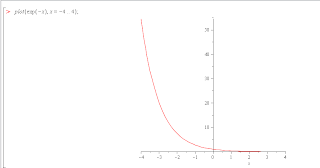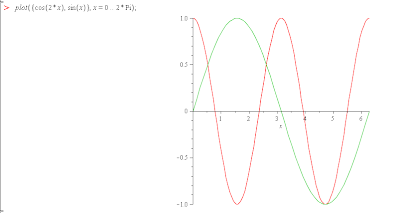## February 20, 2010

### EIS - interface## February 13, 2010

### My PSP...

PSP 3000... he he he## February 4, 2010

### Maple - Lab TestPiloting of function of single variable. Plot graph y=e(-x) with domain (-4 , 4)Plot graph with two variable
y=cos (2x) and y=sin(x) with domain (0,2Pi)

Plot graph circle y=(x^2)+(y^2)## February 2, 2010

### MAPLE 11 - Lab TestUsers can enter mathematics in traditional mathematical notation. Custom user interfaces can also be created. There is extensive support for numeric computations, to arbitrary precision, as well as symbolic computation and visualization. Tomorrow day the first lab test math using software maple.... (^__^)

### Plotting of function of single variable

Plot(sin(x));